# Size and weight of a molecule of oleic acid Estimate of Avogadro's number

(notes by Roberto Bigoni)

## 1. Atoms, molecules and moles.

In the classical Greek culture the philosophical speculation (Democritus, Epicurus, Lucretius in his poem 'De rerum Natura'), relying on the observation of various physical phenomena (the salt solution in water, the evaporation of the water, ...), had assumed that all bodies are composed of tiny invisible particles, not further decomposable, which were then called atoms ('a-tomos' = 'what can not be cut').

The recovery of this hypothesis in the modern era (since the eighteenth century) was at the basis of the birth of the Physics and Chemistry of the structure of matter.

But modern scientists, recovering this hypothesis, withdrew it from the field of metaphysical speculation, to make it subject of scientific inquiry, that is such that its consequences were quantitatively evaluated and experimentally verifiable.

In particular, the chemists of the second half of the eighteenth century became aware of the following facts.

1. Substances seemingly simple (and considered as such for more than two thousand years, like water) could be 'dismantled' in a more simple constituents.

For example water, through electrolysis, can be decomposed into hydrogen and oxygen.

So we can not assume the existence of atoms of water. The smallest particle of water that can be isolated (but this applies in general for many other substances) should be thought of as a particularly stable aggregate of more simple components. The smallest particle of a substance was called moleculeand the name of the atom was reserved to their elementary components.

Only in some rare cases (noble gases) the minimum separable particle of a substance is not further decomposable because it consists of a single atom.

With the development of chemical and physical studies, scientists have understood that there are only 92 kinds of stable atoms, while the number of different types of molecules that can be obtained from combinations of these atoms is practically endless.

2. If, when we decompose a substance (such as water) we get some gases, the ratio between the volumes of the produced gas, in the same conditions of temperature and pressure, is constant and can be expressed in small whole numbers: this is the law of volumes found by the French J. L. Gay-Lussac.

For example, if we decompose a given quantity of water, the volume of hydrogen obtained is twice that of oxygen.

Conversely, if we provoke the formation of water by reacting two volumes of hydrogen with a volume of oxygen, we get two volumes of water (obviously this applies only if also the obtained water is in the gaseous state).

3. This fact has prompted the Italian chemist A. Avogadro to formulate the hypothesis that equal volumes of different gases, under identical conditions of temperature and pressure, contain the same number of molecules.

This hypothesis, after several experimental checks, was taken as one of the foundations of modern science and is now known as Avogadro's principle.

Based on this principle, we can obtain from a given amount of water a number of hydrogen molecules twice the number of oxygen molecules. Conversely, in the formation of water molecules, two hydrogen molecules interact with a molecule of oxygen and from this reaction we get two water molecules.

From the study of this and other chemical reactions, it was understood that the molecules of hydrogen and oxygen are usually composed, respectively, of two atoms of hydrogen and oxygen, and it was natural to represent this phenomenon as follows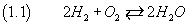4. If we measure the masses of the gases contained in the volumes, we observe that the ratio between the masses, in the same reaction, is constant and can be expressed in small whole numbers; this is the Proust's law.

If we apply this law to the specific case of water we conclude that the mass of a molecule of oxygen is sixteen times that of hydrogen. So the oxygen atom has a mass greater than sixteen times that of hydrogen.

5. The study of many other chemical compounds of hydrogen led the chemists to the conclusion that the hydrogen atom is the one that has the lowest mass.

So it was natural for J. Dalton to assume the mass of the hydrogen atom as mass unit (amu = atomic mass unit), so that all the masses of other atoms and molecules are expressed by an integer multiple of this mass.

The discovery of isotopes, that is of atoms of the same chemical species but of different mass, later led to slightly correct this setting, but, for the purposes of this paper and within the limits of approximations used in the experimental result, it can still be taken as essentially valid.

Then, if we take as the unit mass the mass of a hydrogen atom, the diatomic molecule of hydrogen has mass 2 amu.

6. If we consider 2 g of hydrogen at a temperature of 0°C and a pressure of 1 atmosphere (normal conditions), we can see that this quantity of gas occupies a definite volume (about 22.4 liters). For the Avogadro's principle, this volume is filled by a definite number of molecules.

This number of molecules is called Avogadro's number.

Even if we take 32 grams of oxygen, at equal pressure and temperature, we observe that this quantity of gas occupies the same volume and then must consist of an equal number of molecules.

In general, an amount of substance, made up of as many molecules as there are in 2 g. of hydrogen, is called a mole.

A mole of a given substance, in the gaseous state and under normal conditions, occupy a volume of about 22.4 liters called the molar volume.

In conclusion, to understand how many grams of substance are required to have a mole:

• if the substance is gaseous, we measure the weight of a molar volume;
• otherwise we need to know which and how many atoms compose a molecule of the sustance, then we must calculate the sum of the atomic mass units of their masses (molecular weight) and consider a number of grams equal to the sum.

For example, let we consider the molecule of oleic acid, substance that is liquid at room temperature and is much used in the cosmetic industry to make soaps and creams. It is a compound of 18 carbon atoms, 34 hydrogen atoms and 2 oxygen atoms and therefore is summarily represented as C18H34O2 but better by the following scheme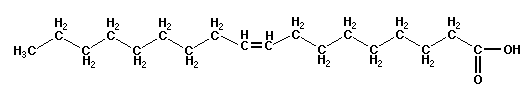If the mass of carbon was 12 amu, that of hydrogen atom 1 amu and that of oxygen atom 16 amu, to have one mole of oleic acid, we should take 282 grams of oleic acid. Due to the existence of isotopes that sum must be corrected in 282.5 grams.

## 2. How estimate the size and the mass of the molecules of a liquid and the Avogadro's number.

It is almost impossible by ordinary means to isolate a single molecule to perform a quantitative study of its properties.

For some substances, however, we can obtain a layer so thin that we can assume that it is almost certainly unimolecular.

For example, Rutherford accomplished his famous experience, which led to the understanding of the internal structure of the atom, by shooting α particles against a thin layer of gold.
But the ability to laminate so thin metal layers requires equipment not usually available.

With liquids the situation is simpler.
If two liquids not miscible and with different densities are in equilibrium in the same container, they form separate layers, with the less dense liquid above the denser one.
The low density liquid, immiscible with water, that will be used in the measurement described later is the oleic acid.

The thickness of each layer depends on the amount of liquid used.

If we use an amount of oleic acid so small as not to allow the formation of a layer completely covering the surface of the water but forms a spot that covers only part of this surface, it seems reasonable to assume that the spot is formed by a unimolecular layer.

If we know the volume V of used acid and and we are able to estimate the surface area S of the spot, we can calculate the thickness d of the spot itself which should reasonably coincide with the thickness of a molecule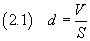If we now assume that the molecule has the form of a cube, we can estimate the volume v of the molecule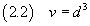Moreover, if we know the density ρ of the oleic acid, we can estimate the mass m of a molecule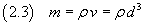Finally, if we know the mass M of one mole of oleic acid, we can estimate Avogadro's number N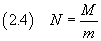## 3. Uncertainties in physical measurements.

The equation (2.1) is purely conceptual, derived from Euclidean geometry, in which a geometrical measurement is given by a number, that will be real and rational or irrational, depending on whether or not it is commensurable with the unit of measure.

In the experimental practice the theoretical value of a measure is never accessible.
The only thing we can do is to establish an upper and a lower bound on the value of this measure, that is, to establish a range within which the value of the measure lies with reasonable certainty.

This becomes very evident if we want to measure the area of the spot of oleic acid.

This area usually has a very irregular shape, that is not comparable with the theoretical figures studied by plane geometry so we can't obtain its measure by measuring lengths of segments.

To estimate this area we can use the following method:
we put a sheet of graph paper under the container holding the liquid;
since liquids are transparent we can see the sheet;
we count the number of squares with a side of 1 cm. completely contained within the perimeter of the spot (q) and then the number of those internal to the spot or crossed by the perimeter of the spot (Q).

After this count, we can say that the area S is in the range [q;Q] cm2.

If we denote by s the half-sum between q and Q and by Δs the half-difference between q and Q, we can also say that the area is in the range [ s-Δs ; s+Δs ] cm2 and express this fact as follows: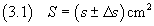The equation (3.1) is an example of the way in which physical measurements are normally expressed.

In the equation (3.1) s is the mean value of the measure, Δs is its absolute uncertainty.

The measure of S is the more accurate the smaller is the ratio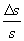This ratio is said relative uncertainty of the measure.
If we would use the same method to obtain a more precise measure, we could count, instead of the squares with sides of 1 cm2 detected by the contour of the spot, the squares with sides of 1 mm2.
The operation would be a little more laborious, but would lead to a much smaller relative uncertainty, that is to a much more precise measure.

## 4. Statistical evaluation of the uncertainty.

A much more meaningful and productive (but more laborious) method to estimate the area S and its uncertainty is the method based on a statistical evaluation.

If we repeat several times the deposition of the same volume of acid on the surface of the water, we will get spots with different measures S.
The quantity S behaves as a random variable. The theoretical analysis of the behavior of random variables carried out by the mathematician K.F. Gauss (1777-1855) leads to the conclusion that the best estimate of the measure is given by the mean value of the distribution of values we have obtained and that the absolute uncertainty is estimated equal to three times its standard deviation

(To quickly obtain the mean value and standard deviation we can use the statistical functions of a spreadsheet).

## 5. Measurement of volume.

Even measuring the volume of the spot requires special precautions.

First, this volume must be very small, otherwise the spot would extend to the entire available surface and we could no longer assume that the layer is unimolecular.

To obtain a sufficiently small volume of oleic acid, instead of directly extract a quantity of acid from its container, we dilute a little of acid in a highly volatile liquid (eg pentane), so that, after the deposition of an amount of solution on the water, the solvent evaporates quickly and only the oleic acid remains on the water. To reduce even more the volume of acid, we can use successive dilutions.

We may proceed as follows:
we prepare a solution of 1 ml of oleic acid in 99 ml of pentane: in this solution there is then 1% of oleic acid;
then we take 1 ml of this solution and we dilute it in 99 ml of pentane: in this second solution there is then 0.1‰ of oleic acid;
therefore, if we take 0.2 ml of this second solution to form the spot, the volume of oleic acid in it is a ten-thousandth of 0.2 ml.

1 ml equals 1 cm3, then the volume of oleic acid we use is 2.10-5 cm3.

Now we must estimate the absolute uncertainty of this measure.
If, for example, to extract this amount of solution we use a pipette graduated in tenths of milliliters and, furthermore, we see that the surface of the liquid in the pipette forms a meniscus, making diffucult to determine the exact level of the liquid, we must attribute to the measurement an uncertainty not less than one-tenth of a milliliter. So we have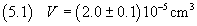## 6. Propagation of uncertainties.

If, for example, with the volume (5.1) we get a spot of acid with area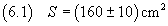we can calculate the measure d of the thickness of the layer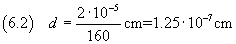But, of course, from uncertain data, we can't obtain a certain measurement.

The uncertainty of this measure can be obtained by applying the rules on the propagation of uncertainties:
in particular, the relative uncertainty of a quotient is given by the sum of the relative uncertainties of its terms, then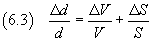So we have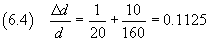The absolute uncertainty is then 0.1125·1.25 10-7 cm, that is about 0.14·10-7 cm.
The order of magnitude of the absolute uncertainty is a tenth of that of the measure, so we consider only the digits with order of magnitude greater than or equal to this, appropriately rounding the measure.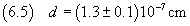Assuming that the oleic acid molecule has cubic form, we can calculate its volume using (2.2).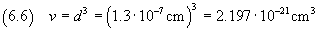The relative uncertainty of the volume is three times the relative uncertainty of the edge, ie 0.3375.

Then the absolute uncertainty is 0.74·10-21 cm3 and the measure of the volume is expressed by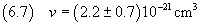The density of oleic acid is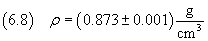therefore, applying the equation (2.3), we can calculate the mass of the molecule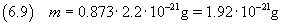The relative uncertainty of a product is given by the sum of the relative uncertainties of its terms. In this case, it practically coincides with the relative uncertainty on the volume (0.3375)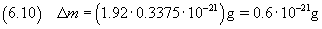So the measure of the mass is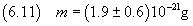we can now estimate Avogadro's number using the equation (2.4)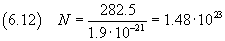The relative uncertainty is still about 0.3375, then the absolute uncertainty is about 0.6·1023

Ultimately we get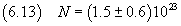The order of magnitude of this value coincides with that obtained with more refined measures but the value of the mantissa is far from satisfactory.

Most likely this discrepancy is due to the fact that we have arbitrarily assumed that shape of the molecule was cubic. Maybe, assuming that other solid geometrical figures approximate better the shape of the molecule, we could obtain better results.

If, for example, we suppose that the shape of the molecule resembles an octahedron consisting of two symmetric pyramids with coincident square bases with side d and both pyramids with height d/2, volume and mass of this octahedron would be one-third of those of the cube considered above, so the evaluations of Avogadro's number and of its uncertainty would be triple than those estimated in (6.13). That is we would obtain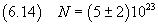Students' works

last revision: October 2015